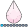## Combining and recoding the variables

Hi,
I have 2 variables: marijuana_Use2 and illegal_drugUse2 both are binary variable.
I want to combine the variables and name as drugUse2, recode as:
0: Never use drug
1: marijuana use
2: Illegal drug use
3: Both drug use
I used the code mention below. I'm able to run the code but I am not sure the outcome I obtain is accurate.

`data myproject7;     set myproject6;    if marijuana_Use2 = 1 and illegal_drugUse2 = 0 then drugUse2 = 1; *Marijuana use;      if marijuana_Use2 = 0 and illegal_drugUse2 = 1 then drugUse2 = 2; *non marijuana illegal drugs use;    if marijuana_Use2 = 1 and illegal_drugUse2 = 1 then drugUse2 = 3; *both drugs use;     if marijuana_Use2 = 1 and illegal_drugUse2 = . then drugUse2 = 1; *Marijuana use;    if marijuana_Use2 = . and illegal_drugUse2 = 1 then drugUse2 = 2; *non marijuana illegal drugs use;    if marijuana_Use2 = 0 and illegal_drugUse2 = 0 then drugUse2 = 0; *never use;    if marijuana_Use2 = 0 and illegal_drugUse2 = . then drugUse2 = 0; *never use;    if marijuana_Use2 = . and illegal_drugUse2 = 0 then drugUse2 = 0; *never use;    label drugUse2 = Drug Use;run;`
5 REPLIES 5HB
Barite | Level 11

## Re: Combining and recoding the variables

Put in a data step and find out

``````data users;
input id mu idu;
datalines;
1105 0 0
1106 0 1
1107 1 0
1108 1 1
1109 . .
1110 . 1
1111 1 .
;
run;

data newcode;
set users;
if mu = 1 and idu = 0 then du = 1; *Marijuana use;
if mu = 0 and idu = 1 then du = 2; *non marijuana illegal drugs use;
if mu = 1 and idu = 1 then du = 3; *both drugs use;
if mu = 1 and idu = . then du = 1; *Marijuana use;
if mu = . and idu = 1 then du = 2; *non marijuana illegal drugs use;
if mu = 0 and idu = 0 then du = 0; *never use;
if mu = 0 and idu = . then du = 0; *never use;
if mu = . and idu = 0 then du = 0; *never use;
label du = Drug Use;
run;``````

results in

 id mu idu du 1105 0 0 0 1106 0 1 2 1107 1 0 1 1108 1 1 3 1109 . . . 1110 . 1 2 1111 1 . 1

which looks right.

You could also go

``````data newcode2;
set users;
du = 0;
if mu = 1 then do;
if idu =1 then du = 3;
else du =1;
end;
if idu = 1 then do;
if mu = 1 then du = 3;
else du =2;
end;
run;``````

just for fun, although I'm not sure it is an improvement.

I would get in the habit of setting a variable like drugUse2 to zero as a default when you create it so every record is populated.

Thank you.

## Re: Combining and recoding the variables

The calculations can be done in one statement. Try:

druguse2 = max(0, marijuanause2)
+ 2*max(0, illegal_druguse2);

## Re: Combining and recoding the variables

One more (untested) variation came to mind:

druguse2 = ^^ marijuanause2 + 2 * ^^illegal_druguse2;

## Re: Combining and recoding the variables

Why not

``druguse2=sum(0,marijuana_use2) + 2*sum(0,illegal_drug_use2);``

The SUM function with zero as one of its arguments as above will produce a zero if the other arguments are missing.

--------------------------
The hash OUTPUT method will overwrite a SAS data set, but not append. That can be costly. Consider voting for Add a HASH object method which would append a hash object to an existing SAS data set

Would enabling PROC SORT to simultaneously output multiple datasets be useful? Then vote for
Allow PROC SORT to output multiple datasets

--------------------------
Discussion stats
• 5 replies
• 259 views
• 0 likes
• 4 in conversation